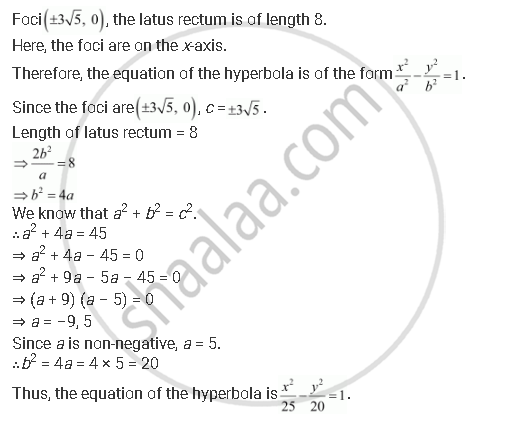CBSE (Science) Class 11CBSE
Share

Books Shortlist

# Find the Equation of the Hyperbola Satisfying the Give Conditions: Foci (+-3sqrt5, 0), the Latus Rectum is of Length 8 - CBSE (Science) Class 11 - Mathematics

ConceptHyperbola Standard Equation of Hyperbola

#### Question

Find the equation of the hyperbola satisfying the give conditions: Foci (+-3sqrt5, 0), the latus rectum is of length 8

#### SolutionIs there an error in this question or solution?

#### APPEARS IN

Solution Find the Equation of the Hyperbola Satisfying the Give Conditions: Foci (+-3sqrt5, 0), the Latus Rectum is of Length 8 Concept: Hyperbola - Standard Equation of Hyperbola.
S